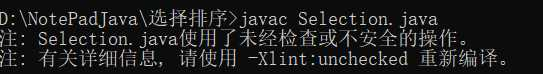# 关于java中“使用了未经检查或不安全的操作、有关详细信息，请使用 ——X临潼：unchecked重新编译”

` 1 public class Selection{  2 public static void main(String[]args){  3 Integer[]a={3,5,7,3,8,27,679,8,564,23};  4  sort(a);  5  show(a);  6  }  7 public static void sort(Comparable<>[]a){  8 for(int i=0;i<a.length-1;i++){  9 int min=i; 10 for(int j=i+1;j<a.length;j++){ 11 if(less(a[j],a[min])) 12 min=j; 13  } 14  exch(a,i,min); 15  } 16  } 17 private static boolean less(Comparable v,Comparable w){ 18 return v.compareTo(w)<0; 19  } 20 private static void exch(Comparable[]a,int i,int j){ 21 Comparable t=a[i]; 22 a[i]=a[j]; 23 a[j]=t; 24  } 25 private static void show(Comparable[]a){ 26 for(int i=0;i<a.length;i++){ 27 System.out.print(a[i]+"  "); 28  } 29  System.out.println(); 30  } 31 }`